# LEAST-SQUARES FINITE ELEMENT ANALYSIS OF FLOWS OF VISCOUS, INCOMPRESSIBLE, GENERALIZED NEWTONIAN FLUIDS

Namhee Kim and J.N. Reddy

## BACKGROUND

### Least-squares finite element model (LSFEM)

• Alternative method to conventional weak-form Galerkin finite element model for fluid problems.
• Minimizing the sum of squared norms of the residuals in governing equations. The basic ides is given below.
• Given an operator equation of the formwe seek suitable approximation of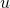as. In the least-squares method, we seek the minimum of the sum of squares of the residuals in the approximation of the equations:### LSFEM’s advantages for Navier–Stokes equations

• Variational framework for the Navier–Stokes equations (minimization problem)

-Free of restrictive compatibility condition.

-Same but higher-order interpolation functions.

• No need a numerical diffusion.
• For linear partial differential equations, symmetric positive-definite (SPD) coefficient matrices regardless of symmetry in the governing equations.

-Linearize the Navier–Stokes equations.

### LSFEM’s deficiencies & remedies

• Higher regularity of the approximate solution; negative effects on the conditioning of coefficient matrices and convergence of solution.

-Equivalent first-order system of governing equations with auxiliary variables (stress or velocity gradient).

• Weak in local mass conservation for both steady and unsteady flow problems.

-Weighting the residual of continuity equation, Higher polynomial order of approximations, Least-squares functional with volumetric flow imbalance.

• Locking problem in low-order LSFEMs for non-H1-coercive formulation in terms of a priori estimate.

-Selective reduced integration methods, High-order approximations, High-order spectral/hp approximations.

### Spectral vs. Lagrange interpolation functions## OBJECTIVE

Develop a least-squares finite element model with high-order spectral/hp approximations for steady flows of generalized Newtonian fluids obeying Carreau–Yasuda viscosity model.

## LEAST-SQUARES FORMULATION

### Least-squares functional(1)### Linearization before minimization

Linearize nonlinear operators in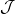: using Newton’s method (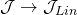)

### Minimization problem ()

Find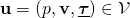, such that(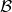: bilinear form,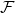: linear form).

## NUMERICAL RESULTS

### Flow over a backward-facing step### Code validation by experimental results (laminar flow) of Armaly et al.(1983)### Parametric study on Carreau-Yasuda fluids: Effect of### Parametric study on Carreau-Yasuda fluids: Effect of### Parametric study on Carreau-Yasuda fluids: Effect of### Lid-driven cavity flowFig. 1. Schematic of the lid-driven cavity flow problem.Fig. 2. Effect of the shape parameter, a, on the viscosity ( 𝑛 = 0.5, 𝜆CY = 1.0 , Re = 100)Fig. 3. Effect of the shape parameter, a, on streamlines on each plane ( 𝑛 = 0.5, 𝜆CY = 1.0 , Re = 100)

### Flow over a backward-facing stepFig. 1: Numerical result of the reattachment length compared with the experimental result by Armaly et al. (1983)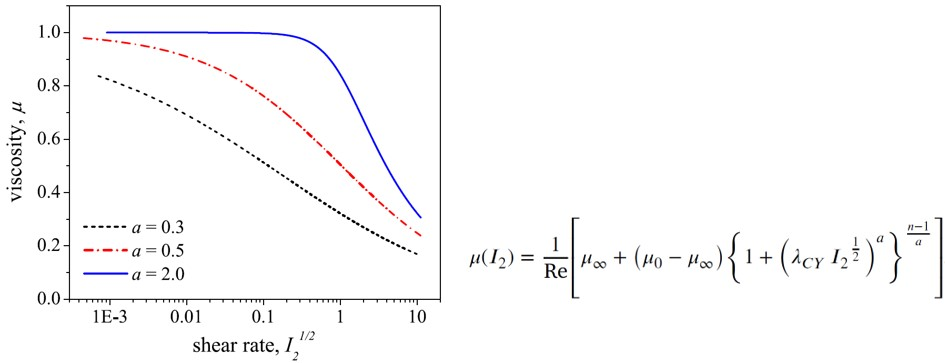Fig. 2. Effect of the shape parameter, a, on the viscosity ( 𝑛 = 0.5, 𝜆CY = 1.0 , Re = 100)Fig. 3. Effect of the shape parameter, a, on streamlines on each plane ( 𝑛 = 0.5, 𝜆CY = 1.0 , Re = 100)

## SUMMARY

• Formulation of a mixed least-squares finite element model (LSFEM) using high-order spectral/hp approximation functions for 2D steady flows of generalized-Newtonian fluids which obey the Carreau-Yasuda viscosity model.
• Numerical example to validate the present numerical model.
• Different effects of the parameters of Carreau-Yasuda model on flow kinematics.

## ACKNOWLEDGEMENT

The authors gratefully acknowledge the support from the Oscar S. Wyatt Endowed Chair account.

## REFERENCE

1. Armaly, B. F., et al. “Experimental and theoretical investigation of backward-facing step flow.” Journal of Fluid Mechanics 127 (1983): 473-496.
2. Kim, Namhee, and J. N. Reddy. “A spectral/hp least‐squares finite element analysis of the Carreau–Yasuda fluids.” International Journal for Numerical Methods in Fluids (2016).